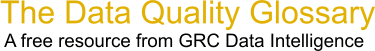Choose Index below for a list of all words and phrases defined in this glossary.

# Sigma Level

index | Index

## Sigma Level - definition(s)

Sigma Level - Determining sigma levels of processes (one sigma, six sigma, etc.) allows process performance to be compared throughout an entire organization, because it is independent of the process. It is merely a determination of opportunities and defects, however the terms are appropriately defined for that specific process.

Sigma is a statistical term that measures how much a process varies from perfection, based on the number of defects per million units.

```   One Sigma = 690,000 per million units
Two Sigma = 308,000 per million units
Three Sigma = 66,800 per million units
Four Sigma = 6,210 per million units
Five Sigma = 230 per million units
Six Sigma = 3.4 per million units
```

In formulae for control limits and process capabilities, sigma is the symbol for Standard Deviation, calculated from the squares of the deviations of measured samples from the mean value (or sometimes by other methods using 'magic' numbers). For a normally distributed output, 99.7% would be expected to fall between +/-(3 x sigma) levels.

[Category=Data Quality ]

Source: iSixSigma, 23 February 2011 09:05:32, http://www.isixsigma.com/index.php?option=com_glossaryData Quality Glossary.  A free resource from GRC Data Intelligence. For comments, questions or feedback: dqglossary@grcdi.nl